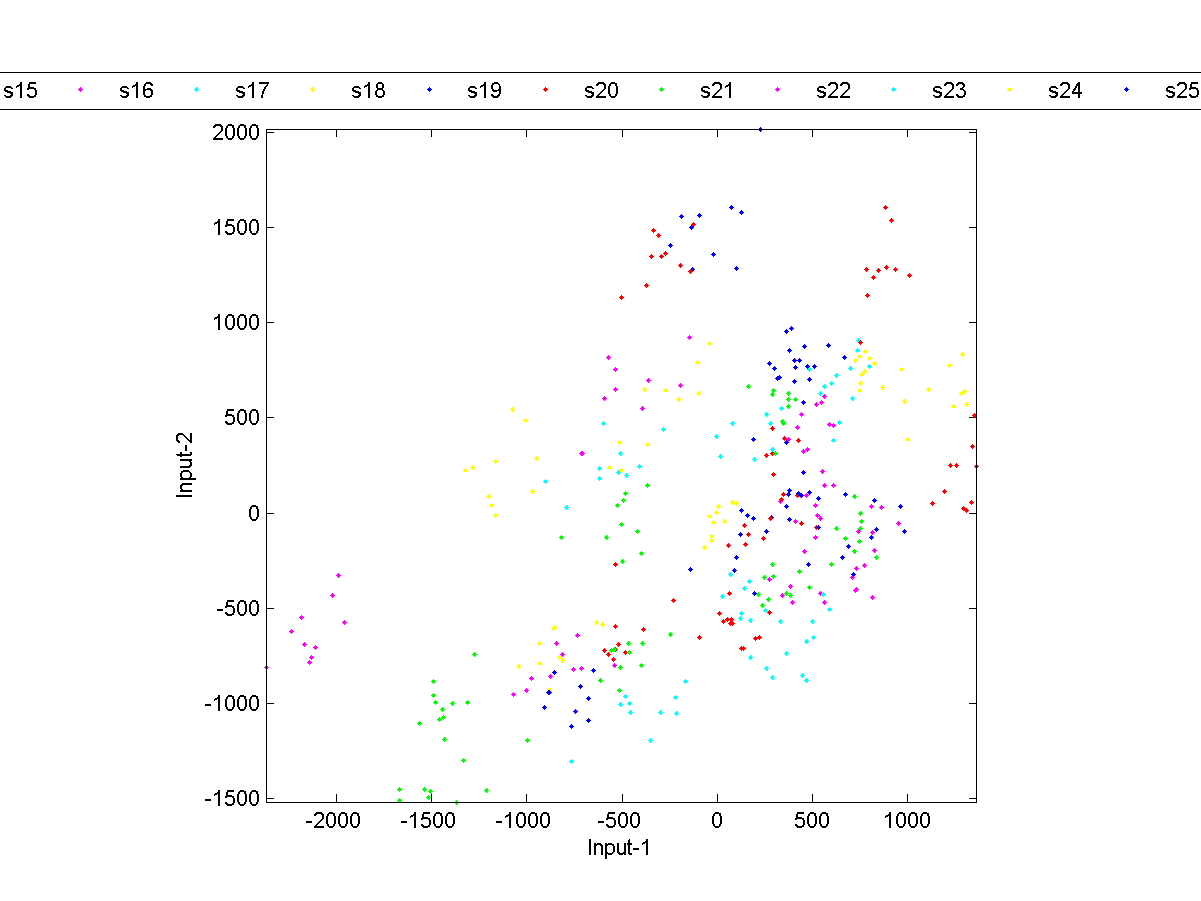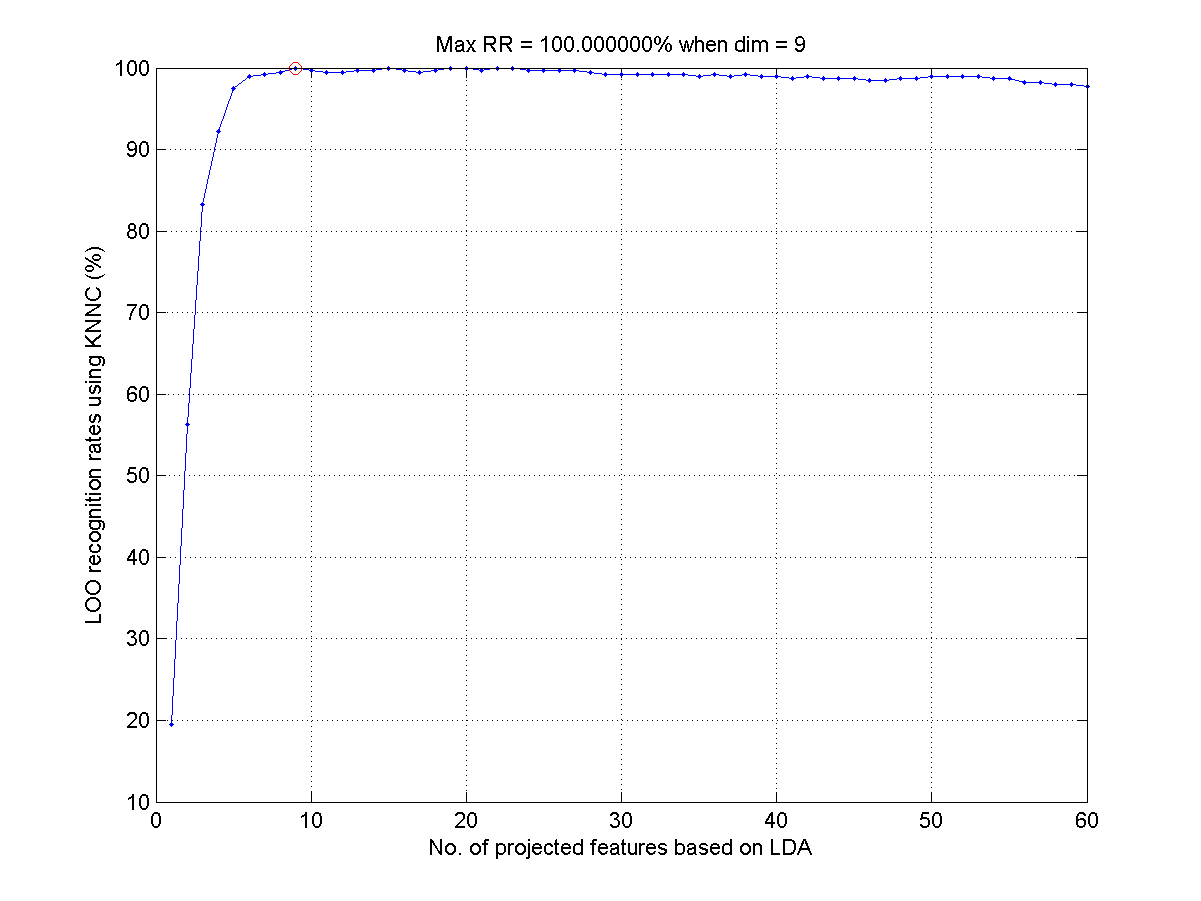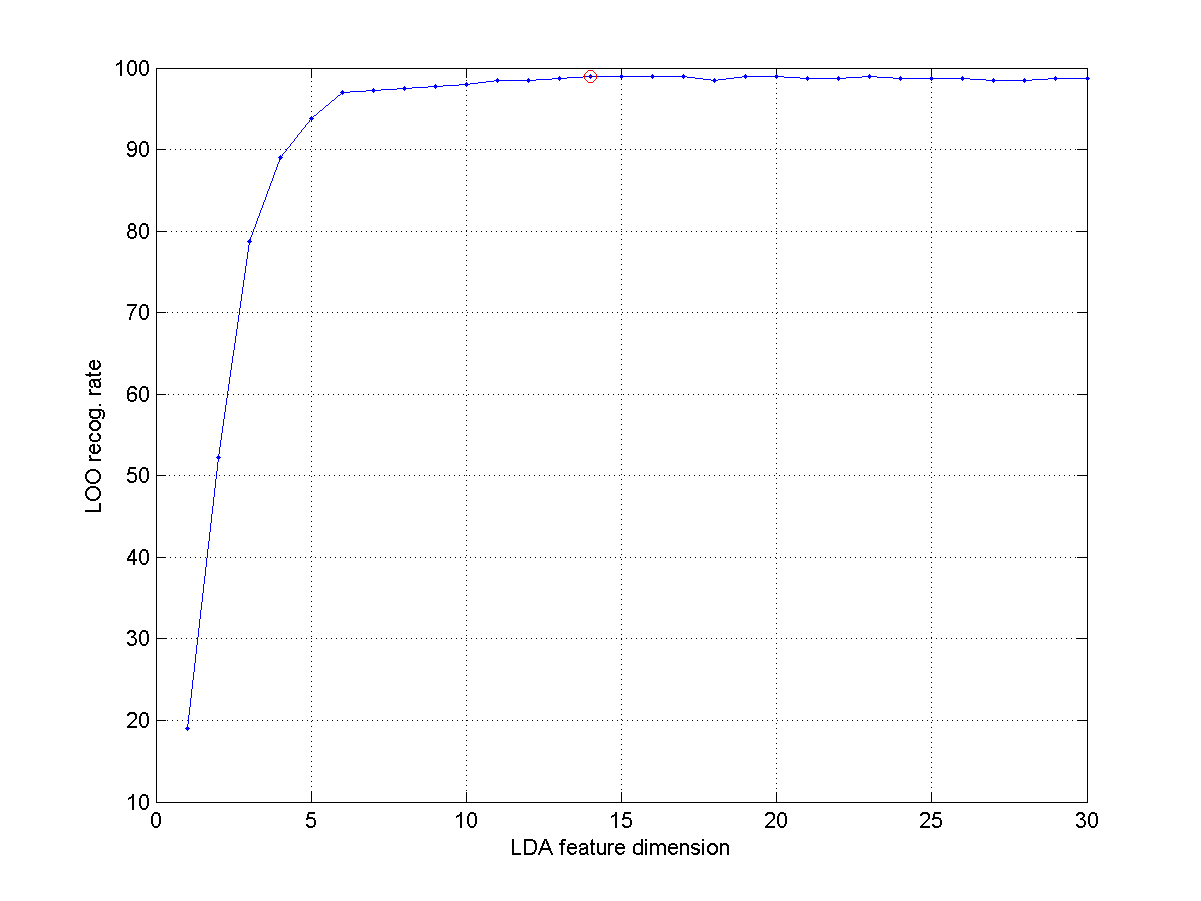11-5 LDA for Face Recognition

In the previous section, we have explain the use of PCA for face recognition, which reduces the feature dimensions from 10304 (=112*92) to 400. The use of PCA can effectively retain the data variance along the first few dimension. However, it does not consider the classes (or identity) of the dataset.

On the other hand, we can apply LDA after PCA for projecting the data along the dimensions with better discriminative power. It should be noted that

• There is no easy way to compute the eigenvectors of LDA using the original 10304 features. As a result, we need to apply PCA first to reduce the dimensions to 400.
• Since the data count is also 400, we cannot use all 400 features for computing the eigenvectors of LDA. (If we use all 400 features, all data points in the same class will be mapped to a single points, leading to a overly optimistic recognition rate of 100%. This is too good to be realistic.)

In the following example, we use the first 60 features after PCA for LDA computation. In order to visualize the data, we select only the first 2 dimensions after LDA for scatter plot:

Example 1: faceRecog/face2dLdaProj01.mload faceData.mat load eigenFaceResult.mat % Load A2, eigVec, rowDim, colDim, etc % ====== Create DS DS.input=A2; DS.outputName=unique({faceData.parentDir}); DS.output=zeros(1, size(DS.input,2)); for i=1:length(DS.output) DS.output(i)=find(strcmp(DS.outputName, faceData(i).parentDir)); DS.annotation{i}=faceData(i).path; end % ====== LDA maxDim=60; DS.input=DS.input(1:maxDim, :); DS2=lda(DS); DS2.input=DS2.input(1:2, :); dsScatterPlot(DS2); [recogRate, computed, nearestIndex]=knncLoo(DS2); fprintf('Recog. rate = %.2f%% after 2D proj. of PCA + LDA\n', 100*recogRate); Recog. rate = 56.25% after 2D proj. of PCA + LDAApparently the classes seem to converge better than PCA alone.

We can vary the dimensions after LDA (and keep the dimensions after PCA to be 60) to see the effects on the overall recognition rate:

Example 2: faceRecog/optLdaEigNum01.mload faceData.mat load eigenFaceResult.mat % Load A2, eigVec, rowDim, colDim, etc % ====== Create DS DS=faceData2ds(faceData); DS.input=A2; % ====== RR w.r.t. no. of eigenvectors myTic=tic; opt.maxDim=60; opt.mode='approximate'; DS.input=DS.input(1:opt.maxDim, :); recogRate=ldaPerfViaKnncLoo(DS, opt, 1); [maxRr, index]=max(recogRate); line(index, maxRr*100, 'color', 'r', 'marker', 'o'); fprintf('Max RR=%.2f%% at dim=%d\n', maxRr*100, index); toc(myTic) LOO recog. rate of KNNC using 1 dim = 78/400 = 19.5% LOO recog. rate of KNNC using 2 dim = 225/400 = 56.25% LOO recog. rate of KNNC using 3 dim = 333/400 = 83.25% LOO recog. rate of KNNC using 4 dim = 369/400 = 92.25% LOO recog. rate of KNNC using 5 dim = 390/400 = 97.5% LOO recog. rate of KNNC using 6 dim = 396/400 = 99% LOO recog. rate of KNNC using 7 dim = 397/400 = 99.25% LOO recog. rate of KNNC using 8 dim = 398/400 = 99.5% LOO recog. rate of KNNC using 9 dim = 400/400 = 100% LOO recog. rate of KNNC using 10 dim = 399/400 = 99.75% LOO recog. rate of KNNC using 11 dim = 398/400 = 99.5% LOO recog. rate of KNNC using 12 dim = 398/400 = 99.5% LOO recog. rate of KNNC using 13 dim = 399/400 = 99.75% LOO recog. rate of KNNC using 14 dim = 399/400 = 99.75% LOO recog. rate of KNNC using 15 dim = 400/400 = 100% LOO recog. rate of KNNC using 16 dim = 399/400 = 99.75% LOO recog. rate of KNNC using 17 dim = 398/400 = 99.5% LOO recog. rate of KNNC using 18 dim = 399/400 = 99.75% LOO recog. rate of KNNC using 19 dim = 400/400 = 100% LOO recog. rate of KNNC using 20 dim = 400/400 = 100% LOO recog. rate of KNNC using 21 dim = 399/400 = 99.75% LOO recog. rate of KNNC using 22 dim = 400/400 = 100% LOO recog. rate of KNNC using 23 dim = 400/400 = 100% LOO recog. rate of KNNC using 24 dim = 399/400 = 99.75% LOO recog. rate of KNNC using 25 dim = 399/400 = 99.75% LOO recog. rate of KNNC using 26 dim = 399/400 = 99.75% LOO recog. rate of KNNC using 27 dim = 399/400 = 99.75% LOO recog. rate of KNNC using 28 dim = 398/400 = 99.5% LOO recog. rate of KNNC using 29 dim = 397/400 = 99.25% LOO recog. rate of KNNC using 30 dim = 397/400 = 99.25% LOO recog. rate of KNNC using 31 dim = 397/400 = 99.25% LOO recog. rate of KNNC using 32 dim = 397/400 = 99.25% LOO recog. rate of KNNC using 33 dim = 397/400 = 99.25% LOO recog. rate of KNNC using 34 dim = 397/400 = 99.25% LOO recog. rate of KNNC using 35 dim = 396/400 = 99% LOO recog. rate of KNNC using 36 dim = 397/400 = 99.25% LOO recog. rate of KNNC using 37 dim = 396/400 = 99% LOO recog. rate of KNNC using 38 dim = 397/400 = 99.25% LOO recog. rate of KNNC using 39 dim = 396/400 = 99% LOO recog. rate of KNNC using 40 dim = 396/400 = 99% LOO recog. rate of KNNC using 41 dim = 395/400 = 98.75% LOO recog. rate of KNNC using 42 dim = 396/400 = 99% LOO recog. rate of KNNC using 43 dim = 395/400 = 98.75% LOO recog. rate of KNNC using 44 dim = 395/400 = 98.75% LOO recog. rate of KNNC using 45 dim = 395/400 = 98.75% LOO recog. rate of KNNC using 46 dim = 394/400 = 98.5% LOO recog. rate of KNNC using 47 dim = 394/400 = 98.5% LOO recog. rate of KNNC using 48 dim = 395/400 = 98.75% LOO recog. rate of KNNC using 49 dim = 395/400 = 98.75% LOO recog. rate of KNNC using 50 dim = 396/400 = 99% LOO recog. rate of KNNC using 51 dim = 396/400 = 99% LOO recog. rate of KNNC using 52 dim = 396/400 = 99% LOO recog. rate of KNNC using 53 dim = 396/400 = 99% LOO recog. rate of KNNC using 54 dim = 395/400 = 98.75% LOO recog. rate of KNNC using 55 dim = 395/400 = 98.75% LOO recog. rate of KNNC using 56 dim = 393/400 = 98.25% LOO recog. rate of KNNC using 57 dim = 393/400 = 98.25% LOO recog. rate of KNNC using 58 dim = 392/400 = 98% LOO recog. rate of KNNC using 59 dim = 392/400 = 98% LOO recog. rate of KNNC using 60 dim = 391/400 = 97.75% Max RR=100.00% at dim=9 Elapsed time is 12.357153 seconds.The recognition rate rises to 100% when the dimension is 9. This indicates how LDA is effective in projecting the dataset along the most discriminative directions. However, there is a caveat here. Since we have used the whole dataset for PCA and LDA, the recognition rate is, again, a little overly optimistic.

In order to evaluate the performance objectively, we need to resort to LOO (leave one out) scheme for face recognition. In other words, when we take a face for test, it cannot be used for computing PCA, LDA, etc. The following example uses such LOO scheme for performance evaluation. (Be warned that it takes hours to run the example.)